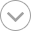Try refreshing the page. If that doesn't work, there may be a network issue, and you can use our self test page to see what's preventing the page from loading.

# Calculus DeMYSTiFieD

ebook

Kindle Book
EPUB ebook

## Languages

English

Calculate this: learning CALCULUS just got a whole lot easier!

Stumped trying to understand calculus? Calculus Demystified, Second Edition, will help you master this essential mathematical subject.

Written in a step-by-step format, this practical guide begins by covering the basics—number systems, coordinates, sets, and functions. You'll move on to limits, derivatives, integrals, and indeterminate forms. Transcendental functions, methods of integration, and applications of the integral are also covered. Clear examples, concise explanations, and worked problems make it easy to understand the material, and end-of-chapter quizzes and a final exam help reinforce key concepts.

It's a no-brainer! You'll get:

• Applications of the derivative and the integral
• Rules of integration
• Coverage of improper integrals
• An explanation of calculus with logarithmic and exponential functions
• Details on calculation of work, averages, arc length, and surface area
• Simple enough for a beginner, but challenging enough for an advanced student, Calculus Demystified, Second Edition, is one book you won't want to function without!